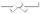# Exercises

0 / 10

For function 'decToBinary', write the missing parts of the recursion case. This function should return a string that stores the binary...0 / 10

Spherical objects, such as cannonballs, can be stacked to form a pyramid with one cannonball at the top, sitting on top of a square...0 / 10

Write a recursive function named 'checkPalindrome' that takes a string as input and returns true if the string is a palindrome and false...0 / 10

Pascal's triangle is a useful recursive definition that tells us the coefficients in the expansion of the polynomial (x + a)^n. Each...0 / 50

Write a recursive function that takes a start index, array of integers,and a target sum, your goal is to find whether a subset of of the...0 / 10

Use appropriate method calls from the List ADT to create the following list: < 4 19| 23 30 > You should assume that L is passed to...0 / 10

Write a recursive function `BSTsmallcount` that, given a BST and a value `key`, returns the number of nodes having values less than `key`. Your...0 / 10

Write a recursive function that returns true if there is a node in the given binary tree with the given value, and false otherwise. Note...0 / 10

Write a recursive function to set the value for each node in a binary tree to be its depth. Assume that nodes store integer values. On...0 / 10

We define a "root-to-leaf path" to be any sequence of nodes in a tree starting with the root node and proceeding downward to a leaf. The...0 / 10

Write a recursive function that returns the sum of the values for all of the nodes of the binary tree with root "root".0 / 10

Given two binary trees, return true if they are structurally identical (they have nodes with the same values, arranged in the same way).

...0 / 10

Use a single assignment statement to make the variable p refer to the Node with info '2' given that the list contains [1,2,3] and points...0 / 10

Given a binary tree, check if the tree satisfies the property that for each node, the sum of the values of its left and right children...0 / 10

Given a binary tree, write a recursive function to return the difference between the sum of all node values at odd levels and sum of all...0 / 10

The height of a binary tree is the length of the path to the deepest node. An empty tree has a height of 0, a tree with one node has a...0 / 10

Write a recursive function to increment by one the value for every node in the binary tree pointed at by "root" then return the modified...0 / 10

Write a recursive function to count the number of leaf nodes in the binary tree pointed at by "root". Assume there is "isLeaf" method to...0 / 10

Given two binary trees, return True if and only if they are mirror images of each other.0 / 10

Given two binary trees, return True if and only if they are structurally identical (ignoring the content of the nodes).0 / 10

Write a recursive function `BSTsmallcount` that, given a BST and a value `key`, returns the number of nodes having values less than `key`. Your...0 / 10

Write a recursive function `BSTsmallcount` that, given a BST and a value `key`, returns the number of nodes having values less than `key`. Your...0 / 10

Write a recursive function `BSTsmallcount` that, given a BST and a value `key`, returns the number of nodes having values less than `key`. Your...0 / 10

Given a binary tree, check if the tree satisfies the property that for each node, the sum of the values of its left and right children...0 / 10

Write a recursive function that returns true if there is a node in the given binary tree with the given value, and false otherwise. Note...